﻿ The Statistical Models Project (SMp) Normal Tissue Complication Probability (NTCP) Model and ParametersPublications are Open
Access in this journal
Article Versions
Export Article
• Normal Style
• MLA Style
• APA Style
• Chicago Style
Research Article
Open Access Peer-reviewed

### The Statistical Models Project (SMp) Normal Tissue Complication Probability (NTCP) Model and Parameters

Terman Frometa-Castillo
American Journal of Applied Mathematics and Statistics. 2017, 5(4), 115-118. DOI: 10.12691/ajams-5-4-1
Published online: November 07, 2017

### Abstract

We provide the most current results of the statistical models project (SMp) related to probabilistic-mechanistic NTCP models for evaluating radiation injury (RI), and discuss some problems of the current radiobiological concepts and models, The SMp NTCP models in function of dose of reference (Dref) were formulated. The SMp (Dref) were used two QUANTEC studies. The SMp will provide of less complex and advantageous models and parameters. Given their unquestionable common elements with the Lyman-Kutcher-Burman (LKB) and sigmoid models derived from logistic functions, they will be applicable; and according to negative remarks pointed out, the well-established, clinically validated, NTCP models in clinical radiobiology, even the SMp NTCP models could replace to these. Until date for fitting real datasets concerning radiation injuries have been used mathematical models that have some similarities with the probabilistic models. All datasets, which have been fitted with mathematical models that have similarities with probabilistic functions (PFs) can also be fitted with PFs.

### 1. Introduction

The tumor control/normal tissue complication probabilities (TCP/NTCP) are probabilistic metrics associated respectively to the efficacies and toxicities in the radiation oncology therapies. Although these therapies are unique where are used probabilistic metrics in the evaluations of their stochastic processes of outcomes, the TCP and NTCP have not been appropriately modeled until date. For example, the current NTCP models are based on sigmoid models.

The logistic functions, which are a popular choice to describe the sigmoid shape dose-response in empirical NTCP models, are non-probabilistic functions (PFs) because: a) They do not define the deterministic regions, and b) They have a double concavity with a double manner of increasing NTCP, before and after their inflexion points. The dose-response does not have sigmoid shape, this is own of the logistic functions. Nonetheless, the usefulness of them is evident and demonstrated during many years. This is conditioned that they have some similarities with the PFs, like NTCP continuously increases in function of its independent variable and takes values in the open interval (0%; 100%).

The SMp proposes a NTCP model function of the dose of reference (Dref), where NTCP continuously increases when Dref increases, and takes values in the closed interval 0%; 100%, and define clearly the stochastic and deterministic regions because NTCP=100% and NTCP=0% in two respective parameters for each determined End-point or complication. These models are used in 1.

Nowadays for evaluating radiation injuries the Lyman-Kutcher-Burman (LKB) NTCP model, sigmoid models derived from logistic functions and the relative seriality model using the Poisson distribution have been used in 2. These are complex mathematical expressions and based on not wholly correct radiobiological-probabilistic foundations.

The SMp has classified the behaviors of the mean values of the stochastic processes/effects (SP/Es) type P1: For values of the independent variable (IV) < threshold value, the process are 0%-deterministic, i.e. it will never occur; for values ≥ this threshold, the process is increased when the IV increases, from 0% to 100%, and is stochastic until a determined value of IV where the process becomes 100%-deterministic, i.e. it certainly occurs.

This study proposes NTCP models, which do not involve dose nor volume values from dose-volume histograms (DVHs), but some metrics characterizing to them, like maximum or mean dose, as dose of reference (Dref) in the SMp NTCP(Dref).

### 2. The SMp NTCP Model Function of a Dose of Reference (Dref)

Based on the SMp type P1, it has been formulated new probabilistic-mechanistic NTCP models, like for most critic End-Points, i.e. Grade Xmax (Xmax: maximum value of X) or global complication, i.e. Grade ≥ X of an OAR (Organ at risk), the SMp NTCP(Dref) model as: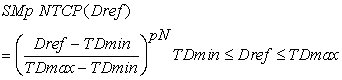(1)

where, TDmin: Threshold value of Dref for an End-Point with Grade ≥ X

TDmax: Minimum value of Dref for NTCP=100%;

pN: Power of this SMp NTCP model (pN>0)

Dref: Dose of reference, such as maximum dose (Dmax) or mean dose (Dmean) in the normal tissue DVH.

In Dref< TDmin and Dref>TDmax the normal tissue complications is a respectively 0% and 100% deterministic process

Note: For multiple OARs, NTCP= max (NTCPT), where NTCPT is NTCP for each individual OAR or tissue T.

### 3. The Standardized NTCP Models

3.1. The Lyman-Kutcher-Burman (LKB) NTCP Model and Other Ones Using Logistic Functions

The origin of some deficiencies concerning the current NTCP evaluations was the use of LKB NTCP model, a normal cumulative distribution function (CDF), which is not a probabilistic function, but is just that its name indicates. The sigmoid models derived from logistic functions have the same mathematical behavior that LKB has.

The dose-response does not have a sigmoid shape, it has been associated with this shape; because this is own of the LKB and logistic functions, which have habitually been used for fitting these responses.

The current sigmoid NTCP model is a curve with double concavity. Before its inflexion point is a concave upward, while after this is a concave downward. For this model the manner of NTCP increases in function of its independent variable in both concavities is different.

The widely used LKB NTCP model is mathematically and computational complex. This has three parameters (TD50, m and n of the Deff expression). This involves three equations: Eq. (2)-(4). The EUD-based NTCP model 3, 4 also has three parameters (TD50, γ50 and a of EUD expression). Our proposing SMp NTCP(Dref) models use directly as referential dose one of the metrics characterizing the DVHs, instead EUD or Deff approaches.

The LKB model is expressed in 2 as: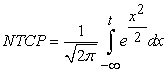(2)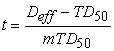(3)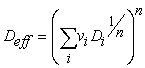(4)

Here, the µ is taken as TD50 and σ as m*TD50. Deff is the dose that if given uniformly to the entire volume, will lead to the same NTCP as the actual non-uniform dose distribution, TD50 is the uniform dose given to the entire organ that results in 50% complication risk and m is a measure of the slope of the sigmoid curve. The vi represents the ith partial volume receiving dose Di.

The LKB model is a sigmoid curve. This has an inflexion point (IP) with a rapid increase for values of the Deff<= value of the IP, and slow increase for other values of Deff. Given the sigmoidal behavior is not an acceptable radiobiological behavior, you should not use the CDF for characterizing probabilities of stochastic processes, except in the random generation of the stochastic independent variables in the Monte Carlo Simulation. For this reason, additional to its high mathematical complexity, despite the fact that the LKB NTCP is a phenomenological model, is not a proper probabilistic-radiobiological model, but a pure mathematical one.

The sigmoid models derived from logistic functions have lees complex mathematical expressions than LKB, but they follow the same sigmoidal behavior.

In the SMp NTCP(Dref) model, NTCP continuously increases when Dref increases, and takes values in the closed interval 0%; 100%, it does not have inflexion points, and clearly define the deterministic regions because NTCP=100% in Dref=TDmax and NTCP=0% in Dref=TDmin for each determined End-point.

The SMp NTCP(Dref) model has some similarities to the NTCP models derived from logistic functions. Both continuously increase in function of their independent variables, and NTCP takes values in the opened interval (0%, 100%), but our proposal does not have IP, and has well-defined boundaries of the non-stochastic and stochastic regions. The sigmoid model has an IP with a rapid increase for values of the EUD or Deff <= value of the IP, and slow increase for other values of EUD or Deff, which is not an acceptable radiobiological behavior. For this reason, you should not use these for characterizing probabilities of stochastic processes. Additional to its high mathematical complexity, despite the fact that quoted models are phenomenological, they are not proper probabilistic-radiobiological functions.

The SMp NTCP models could be used in some QUANTEC studies as mathematic/biologic models, like in 5 where the association between radiation pneumonitis (RP) risk and the mean lung dose (MLD) was expressed by a logistic function (LF) as: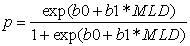(5)

where, b0 and b1: Mathematical parameters

MLD: The mean lung dose

p: Represents the RP risk

This function has the following deficiencies: a) It is very similar to the LKB NTCP model, but with less mathematical complexity. Both non-probabilistic models do not define the deterministic regions, and have the same unreal conditions for RP=100% and RP=0%, respectively in MLD= positive infinity and MLD= negative infinity; b) Moderate grade of mathematic complexity; and c) Its two parameters (b0 and b1) are purely mathematical. The other deficiency related to double concavity is not shown because the fit for region occupied by the data was done with the concave-upward part of the LF.

If the LF is replaced by the SMp NTCP(MLD) the quoted deficiencies will disappear because: a) This is purely a probabilistic model, which clearly defines the limits of the non-stochastic regions; b) Low grade of mathematic complexity; and c) Two of its three parameters are radiobiological concepts. An acceptable-fit SMp NTCP(MLD) parameters to the data in figure 2(a) of 5 are: TDmin= 10 Gy, TDmax= 50 Gy and pN= 1.17.

For data described by the LKB NTCP(Deff) model in 6 with TD50=65Gy, m=0.14, n=0.25 (Deff≈Dmax), an acceptable-fit is done with the following SMp NTCP(Dmax) parameters: TDmin=45Gy, TDmax=85Gy, and p=1.1.

The sets of the SMp parameters, as whatever ones, are associated to a determined treatment circumstance (spatial and temporal dose distribution, smoking status, chemotherapy, etc.)

Until date, the datasets have been acceptably fitted with mathematical functions (MFs), like LKB that have some similarities with the probabilistic functions (PF). If you use a PF, like the SMp NTCP(Dref) the fit is more acceptable. You can get the SMp parameters using the graphical/analytical tools developed by this study, and the parameters obtained with the quoted MFs.

3.2. Relative Seriality Model

The relative seriality model (RSM) or the s-model describes response of an organ with a mixture of serial- and parallel-arranged functional subunits (FSUs). The relative contribution of each type of architecture is described by the parameter s, which is equal to unity for a fully serial organ and zero for a fully parallel organ. NTCP is given by the following equation: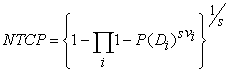(6)

This is premised on a simple argument that, if the probability of failure for a single FSU, p, is known, the probability of failure for an entire organ composed of N FSUs arranged in a series can be calculated as 1 – (1 –p)N, where vi is the fractional organ volume receiving a dose Di and P(Di) is the complication 2.

This model does not have a solid-probabilistic foundation because of this is premised on an incorrect argument. The correct for N FSUs arranged in a series is expressed in the following equation: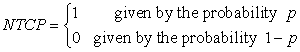(7)

The usefulness of this model is given: 1- NTCP takes values in the open interval (0%; 100%); and 2- It continuously increases when P(Di) increases, i.e. Di increases. Additional to the mathematical complexity, its deficiencies also are: the values of P(Di) are determined using the Poisson model – an exponential function- , this does not define the deterministic regions because NTCP=100% in P(Di)=100%, i.e. Di=positive infinity. This condition is wholly out of the reality, and it is the same for whatever End-point. According to deficiencies previously pointed out to the Eq. (6), the RSM is non-probabilistic function, and when is used the Poisson distribution for its calculations, then it also is an improper model.

### 4. Conclusion

Given our probabilistic SMp NTCP (Dref) model are not sigmoid curves, i.e. it has no inflexion point, and unquestionable common elements with the Lyman-Kutcher-Burman (LKB) NTCP and other sigmoid models derived from logistic functions, this will be applicable in radiation oncology therapy; and according to negative remarks pointed out to the well-established, clinically validated, NTCP models in clinical radiobiology, even the SMp NTCP models could replace to these.

Until date for fitting real datasets concerning radiation injuries have been used mathematical models (MMs) that have some similarities with the probabilistic functions. The LKB NTCP model and logistic functions are not wholly appropriate for describing the NTCP. Never a MM will do a better fit to datasets of mean outcomes of stochastic processes than a probabilistic one.

All datasets, which have been fitted with MMs that have similarities with probabilistic functions (PFs), can also be fitted with these.

The dose-response does not have a sigmoid shape, it has been associated with this shape; because this is own of the LKB and logistic functions, which have habitually been used for fitting these responses.

Although the results reported by the SMp NTCP will be not very different to those calculated by the LKB NTCP model or other sigmoid ones, the use of the SMp will allow:

• Avoiding the conceptual error of using the LKB NTCP model (the normal cumulative distribution function) and other sigmoid models in evaluating probabilities

• Using a model with much less mathematical complexity

• Using two radiobiological parameters and one mathematical more advantageous than the mathematical parameters of the quoted sigmoid models.

• Using the same type of model that will be used for other stochastic processes and effects of the radiation oncology therapies.

The proposed SMp NTCP(Dref) model does not involve approaches derived by effective dose (Deff) nor equivalent uniform dose (EUD), but directly metrics that characterize to dose-volume histograms, such as maximum dose (Dmax) and mean dose (Dmean). The SMp NTCP model will be used for serial or parallel organs, but their parameters should be associated to a determined treatment circumstance.

According to deficiencies pointed out, the relative seriality model is a non-probabilistic function, and when is used the Poisson distribution for its calculations, also it is an improper model.

### Acknowledgements

The most sincere acknowledgement to the Dr. Raul Piseaux-Aillon (Co-Founder of the SMp) for his appreciable contributions and advices.

### References

  Jang S., Frometa-Castillo T., Pyakuryal A., Sio T.,et al., “The Statistical NTCP and TCP models in the Proton therapy”; Med. Phys. 43, 3451. 2016. In article View Article  Report of AAPM TG 166, “The Use and QA of Biologically Related Models for Treatment Planning”. 2012. In article View Article  Niemierko A., Goitein M., “Calculation of normal tissue complication probability and dose volume histogram reduction schemes for tissues with a critical element architecture”, Radiother Oncol. 20(3):166-176, 1991. In article View Article  Gay H.A., Niemierko A., “A free program for calculation EUD-based NTCP and TCP in external beam radiotherapy”, PhysicaMedica 23, 115-125, 2007. In article View Article  PubMed  Marks L.B., Bentzen S.M., Deasy J.O., et al., “Radiation dose-volume effects in the lung”, Int J. Radiation Oncology Biol. Phys. Vol. 76 No. 3, Supplement, pp. S70-S76, 2010. In article View Article  PubMed  Mayo C., Martel M.K., Marks L.B.. et al., “Radiation dose-volume effects of optic nerves and 474 chiasm”, Int J. Radiation Oncology Biol. Phys. Vol. 76 No. 3, Supplement, pp. S28-S35, 475 2010. In article View ArticleThis work is licensed under a Creative Commons Attribution 4.0 International License. To view a copy of this license, visit http://creativecommons.org/licenses/by/4.0/